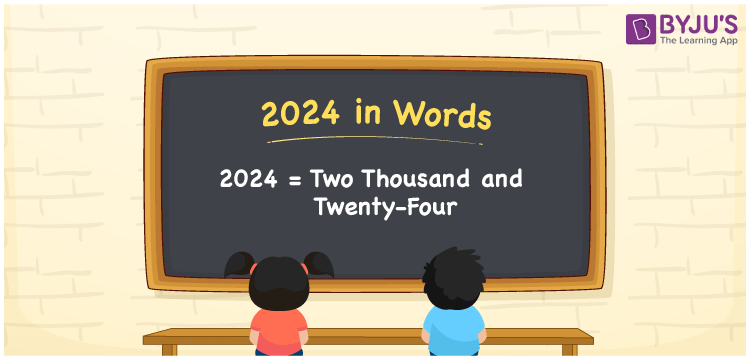# 2024 in Words

The number 2024 in words is “two thousand and twenty-four”. If you have purchased the stationery items worth Rs. 2024”, you can write it as “I have purchased the stationery items worth Rs. Two thousand and twenty four”. Also, the number 2024 is a cardinal number

 2024 in Words: Two Thousand and Twenty-four. Two Thousand and Twenty-four in Numerical Form: 2024.

## 2024 in English Words## How to Write 2024 in Words?

Go through the below table to learn the place values of 2024:

 Thousands Hundreds Tens Ones 2 0 2 4

The expanded form of 2024 is as follows:

= 2 × Thousand + 0 × Hundred + 2 × Ten + 4 × One

= 2 × 1000 + 0 × 100 + 2 × 10 + 4 × 1

= 2000 + 20 + 4

= 2024

= Two thousand and twenty-four

Hence, 2024 in words is two thousand and twenty-four.

2024 in words – Two thousand and twenty-four

Is 2024 an odd number? – No

Is 2024 an even number? – Yes

Is 2024 a perfect square number? – No

Is 2024 a perfect cube number? – No

Is 2024 a prime number? – No

Is 2024 a composite number? – Yes

## Frequently Asked Questions on 2024 in Words

Q1

### How to write 2024 in words?

2024 in words is two thousand and twenty-four.

Q2

### Simplify 2000 + 24, and express it in words.

Simplifying 2000 + 24, we get 2024. Hence, 2024 in words is two thousand and twenty-four.

Q3

### Is 2024 an even number?

Yes, 2024 is an even number.